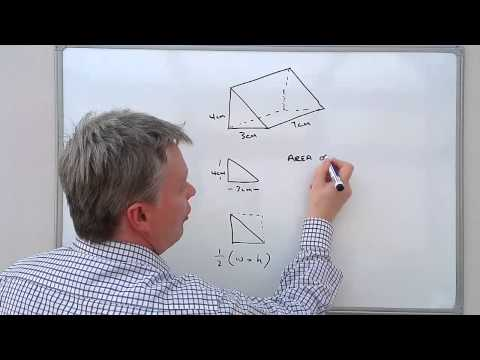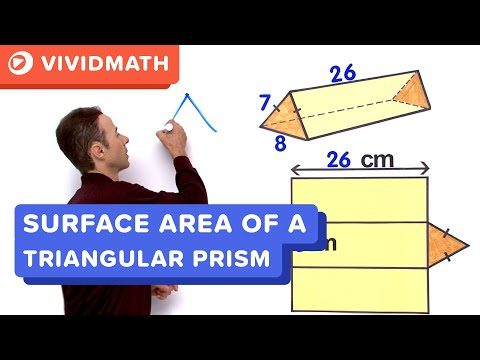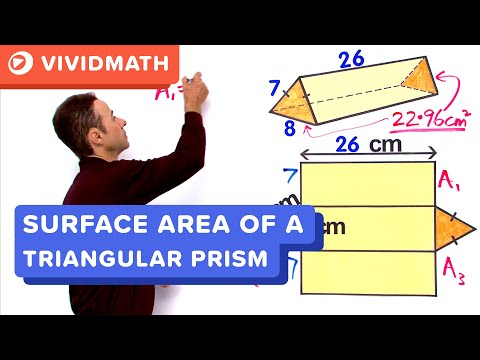## Triangular prism

Subject: Compulsory Mathematics

#### Overview

A triangular prism is a prism composed of two triangular bases and three rectangular sides. A prism with the triangle as the base is called triangular prism. In case of triangular prism, two congruent and parallel triangles

##### Triangular prism#### Volume and surface area of Triangular prism

A prism with the triangle as the base is called triangular prism. In the case of a triangular prism, two congruent and parallel triangles ABC and EFG are called the base of the prism. Area of each triangle is called base area or area of the base.

The lateral faces (AEFB, AEGC and BCGF), are rectangles formed by joining corresponding vertices of the bases. The intersection of lateral faces is lateral edges.

The length or height  ( AE, BF, CG ) is the perpendicular distance between the bases. The lateral surface is the total area of the lateral faces (The length times the perimeter of base) and volume is equal to the product of base area and its length or height.

So,

Area of triangular base = Area of  ΔABC or Area of ΔEFG

Lateral (Curved) Surface Area (L.S.A) = Perimeter of triangular base × lengthTotal Surface Area (T.S.A) = 2 × Area of triangular base + L.S.A.

Volume of triangular prism = Area of triangular base × length (or height of prism)

##### Things to remember

Area of triangular base = Area of  ΔABC or Area of ΔEFG

Lateral (Curved) Surface Area (L.S.A) = Perimeter of triangular base × length

Total Surface Area (T.S.A) = 2 × Area of triangular base + L.S.A.

Volume of triangular prism = Area of triangular base × length (or height of prism)

• It includes every relationship which established among the people.
• There can be more than one community in a society. Community smaller than society.
• It is a network of social relationships which cannot see or touched.
• common interests and common objectives are not necessary for society.
##### Videos for Triangular prism##### How to work out the volume of a triangular prism##### Surface Area of a Triangular Prism##### Surface Area of a Triangular Prism PT2 - VividMaths.com

Solution:

Here,

\begin{align*}\text{base area of prism (A)} &= \frac{1}{2} \times base \times height \\ &= \frac{1}{2} \times 6 \times 8 \\ &= 24 \: cm^2 \end{align*}

height of prism (h) = 30 cm
By formula,

$Volume\: of \: prism (V) = A\times h = 24 \times 30 = 720\:cm^2 \: \: _{Ans}$

Solution:

BC = B'C' = 5 cm

\begin{align*} \text{Perimeter of the base triangle} &= AB+BC+AC\\ &= 3cm+5cm+AC \\ &= 8cm+AC \\ Height \: of\: the \: prism (h) &= 20\: cm \\ Rectangular \: surface \: area \: of\:prism &=ph \\ or, 240cm^2 &= (8cm + AC) .20cm \\ or, 8cm + AC &= \frac{240cm^2}{20cm}\\ or, 8cm+AC &= 12cm \\ or, AC &= 12cm-8cm \\ \therefore AC &= 4 cm \: _{Ans}\end{align*}

Solution:

\begin{align*} 2s &= PQ+PR+QR \\or, 2s &= (6+7+5)cm \\or,2s &= 18 cm \\ or, s&=\frac{18}{2}\\ \therefore s &= 9cm \end{align*}

Now,

\begin{align*} Area \: of \: \Delta PQR &= \sqrt{s(s-a)(s-b)(s-c)}\\ &= \sqrt{9 (9-6)(9-7)(9-5)}cm^2\\ &= \sqrt{9\times3\times2\times4}cm^2 \\ &= \sqrt{216}cm^2 \\ \: \\ Volume \: of \: prism &= A \times height \\ &= \sqrt{216}\times18 \: cm^3 \\ &= 264.54 \: cm^3 \end{align*}

Solution:

V = Volume of prisms = 864 cm3

$A = \text{Area of rt. angled triangle }= \frac{1}{2}\times 8 \times 9 = 36cm^2$

H = height of prism = ?

By formula, we have

\begin{align*} V &= A \times h \\ or, 864cm^3 &= 36cm^2 \times h \\ or, h &= \frac{864cm^3}{36cm^2} \\ \therefore h &= 24cm _{ans} \end{align*}

Solution:

Here, AE =10cm, AF = BC = 8cm

$EF = \sqrt{AE^2 - AF^2 } = \sqrt{10^2 - 8^2} = \sqrt{36} = 6 cm$

$\text{Perimeter of base triangle} = 10cm + 8cm+6cm = 24 cm$

height (h)= 20cm

\begin{align*} \text{Lateral surface area } \: &= P \times h \\ &= 24 cm \times 20 cm \\ &= 480 cm^2 \: \: \: _{Ans}\end{align*}

Solution:

Here,

$P = AB + BC + CA \\ \: \: \: = 2\sqrt{3} + 2\sqrt{3} + 2\sqrt{3}\\ \: \: \: = 6\sqrt{3} cm$

\begin{align*} \text{Area of rectangular surface}&= P \times CK \\ &= 6\sqrt{3}\times 4\sqrt{3}\\ &= 72cm^2 \: _{Ans} \end{align*}

Solution:

\begin{align*} A&=Area \: of \: base \\ &=area \: of \: rt. \: angled \: \Delta ABC \\ &= \frac{1}{2}\times BC \times AB \\ &= \frac{1}{2}\times 5cm \times 12 cm \\ &= 30 \: cm^2 \\ In \: ABC, \\ AC^2 &=AB^2+BC^2\\ or, AC&=\sqrt{(12cm)^2 + (5cm)^2}\\ or, AC &= \sqrt{144cm^2+25cm^2} \\ \therefore AC &= \sqrt{169cm^2} = 13cm \\ \: \: \\ P = Perimeter \: of \: \Delta &= AB+BC+AC \\ &= 12cm+5cm+13cm\\ &= 30 cm \\ \: \\ S= Lateral\:surface\:area&= P\times h\\ &= 30cm\times 30cm\\ &= 900cm^2 \\ \: \\ Total \: surface \: area&= 2A + S \\ &=2 \times 30cm^2 + 900cm^2\\ &= 960cm^2 \: \: _{Ans} \end{align*}

Solution:

\begin{align*} The \:area (A) \: of \: the \: base &= l^2\\ &= (6cm)^2 \\&=36cm^2 \\ Perimeter (P)\: of \: of \: the \: base &= 4l \\ &=4 \times 6 \\ &=24cm \\ The \: height(h) of \: the\:prism&=12 \\ Here, \:\:\:\:\:\:\:\:\:\:\:\:\:\:\:\:\:\:\:\:\:\:\:\:\:\:\:\:\:\:\:\:\:\:\:\:\:\:\:\:\:\:\: \\ Total\:surface\:area &= 2 \times area \: of \: base + L.S.A \\ &= 2A + Ph\\ &= 2\times36+24\times12 \\ &= 72cm^2+288cm^2 \\ &= 360cm^2 \end{align*}

Solution:

Let h be the height of the prism.
Here, Volume = 48cm3

Area of base triangle (A) =?

\begin{align*} A &= \frac{1}{2} \times 4 \times 3 \\ &= 6 \: cm^2 \\ By \: formula, \\ Volume &= A \times h \\48^3 &= 6cm^2 \times h \\ or, h &= \frac{48cm^3}{6cm^2} \\ \therefore h &= 8 cm \: \: _{Ans} \end{align*}

Solution:

Length of the side of the base (a) = 6 cm

\begin{align*}Area \: of \: base\: triangle\: (A)&= \frac{\sqrt{3}}{4}a^2 \\ &= \frac{\sqrt{3}}{4}6^2 \\ &= 9\sqrt{3} \: cm^2 \\ Volume(V) &= 162 \: cm^3\\ height \: of \: the \: prism \: (h)&= ? \\ We \: know \: that, \: \: \:\:\:\:\:\:\:\:\:\: \\ V&=A \times h \\ 162 \:cm^3&=9\sqrt{3} \times h \\ or, h &= \frac{162}{9\sqrt{3}} \\ or, h &= 6\sqrt{3} \\ \therefore h &= 10.39 \: cm \end{align*}

Solution:

\begin{align*} Perimeter \: of \: base \: triangle \: (P) &= AB+BC+AC \\ &= 3cm+5cm+4cm\\&= 12cm\\ Height \: (h) \:\:= CC' &= ? \\ By, formula, area \: of \: rectangular \: faces &= Ph \\ or, 240 \: cm^2 &= 12cm\times h \\ or,h&=\frac{240cm^2}{12cm}\\ \therefore h &= 20cm \: _{Ans} \end{align*}

Solution:

Perimeter of base (P) = ?
Rectangular surface area (S) = 600 cm2
Height of prism (h) = 32 cm
By formula,

\begin{align*} S &=Ph \\ or, 660&=P\times 22 \\ or, P &= \frac{660}{22}\\ \therefore P &= 30 \: cm \: _{Ans} \end{align*}

Solution:

Here, V = 450 cm3 , h = CD = 15 cm, AC = ?

By formula,

\begin{align*} V&=Ah\\ or, A &= \frac{V}{h}\\ &= \frac{450}{15}\\ \therefore A &= 30 \: cm^2 \\ Again \: by \: the \: formula, \\ A&=\frac{1}{2}AB \times BC \\ or, 30 &= \frac{1}{2}\times 12 \times BC \\ or, 30 &= 6BC \\ or, BC &= \frac{30}{6}\\ \therefore BC &= 5 \: cm \\ From \: right \: angled \: &triangle \: ABC, \\ AC^2 &= AB^2 + BC^2 \\ or, AC^2&= 12^2 + 5^2 \\ or, AC&=\sqrt {169} \\ \therefore AC &= 13 \: cm \: _{Ans} \end{align*}

Solution:

Here,

\begin{align*} BC &= \sqrt{AC^2-AB^2}\\ &=\sqrt{(20cm)^2 - (12cm)^2}\\ &=\sqrt{400-144}cm \\ &= \sqrt{256}cm \\ &= 16cm \\ \: \\ \text{Area of right angled} \: &triangle \: of \: base \: (A) = \frac{1}{2}\times AB \times BC \\ &= \frac{1}{2} \times 12 \times 16 \\ &= 6cm \times 16cm \\ &= 96cm^2 \\ \: \\ Suppose, height \: of \: the \: prism &= h \\ Then, volume \: of \: the \: prism &= Ah \\ or, 1920cm^3 &= 96cm^2\times h \\ or, h &= \frac{1920cm^3}{96cm^2}\\ \therefore h &= 20 cm \: _{Ans} \: cm \end{align*}